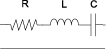# seriesRLC

Create two-port series RLC circuit

## Description

Use the `seriesRLC` object to create a circuit representing as a resistor, inductor, and capacitor connected in series. The series RLC circuit object is a two-port network as shown in this circuit diagram.You can also use the `seriesRLC` object to model a series RLC circuit in cascaded stages using an `rfbudget` object or the RF Budget Analyzer app.

## Creation

### Syntax

``rlc = seriesRLC``
``rlc = seriesRLC(Name,Value)``

### Description

````rlc = seriesRLC` creates a default series RLC object.```

example

````rlc = seriesRLC(Name,Value)` sets Properties of the `serisRLC` object using one or more name-value arguments. For example, `rlc = seriesRLC('R',80)` creates a series RLC object with the resistance set to `80` ohms. Properties you do not specify retain their default values.```

## Properties

expand all

Name of the series RLC circuit, specified as a string scalar or a character vector.

Example: `rlc = seriesRLC('Name','RLCseriescircuit')` creates a series RLC circuit called `RLCseriescircuit`.

Resistance value of the series RLC circuit, specified as a positive scalar in ohms.

Example: `rlc = seriesRLC('R',75)` creates a series RLC circuit with the resistance set to `75` ohms.

Inductance value of the series RLC circuit, specified as a positive scalar in henries.

Example: `rlc = seriesRLC('L',1e-6)` creates a series RLC circuit with the inductance set to `1e-6` henries.

Capacitance value of the series RLC circuit, specified as a positive scalar in farads.

Example: `rlc = seriesRLC('C',2.2e-9)` creates a series RLC circuit with the capacitance set to `2.2e-9` farads.

Number of input and output ports of the series RLC circuit, returned as a positive scalar.

Terminals of the series RLC circuit, returned as a cell array.

## Object Functions

 `sparameters` Calculate S-parameters for RF data, network, circuit, and matching network objects `groupdelay` Group delay of S-parameter object or RF filter object or RF Toolbox circuit object `noisefigure` Calculate noise figure of transmission lines, series RLC, and shunt RLC circuits

## Examples

collapse all

Create a series RLC circuit with the specified parameters.

`rlc = seriesRLC('R',2e3,'L',40e-3,'C',1e-6);`

Calculate the S-parameters of the series RLC circuit at `1` GHz.

`spar = sparameters(rlc,1e9)`
```spar = sparameters: S-parameters object NumPorts: 2 Frequencies: 1.0000e+09 Parameters: [2x2 double] Impedance: 50 rfparam(obj,i,j) returns S-parameter Sij ```

Calculate the noise figure of the series RLC circuit at `1` GHz.

`nf = noisefigure(rlc,1e9)`
```nf = 16.1278 ```

## Version History

Introduced in R2021a